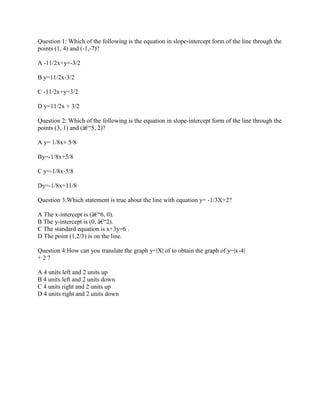Seu SlideShare está sendo baixado. ×

# Question 1- Which of the following is the equation in slope-intercept.docx

Anúncio
Anúncio
Anúncio
Anúncio
Anúncio
Anúncio
Anúncio
Anúncio
Anúncio
Anúncio
AnúncioCarregando em…3
×

1 de 2 Anúncio

# Question 1- Which of the following is the equation in slope-intercept.docx

Question 1: Which of the following is the equation in slope-intercept form of the line through the points (1, 4) and (-1,-7)?

A -11/2x+y=-3/2

B y=11/2x-3/2

C -11/2x+y=3/2

D y=11/2x + 3/2

Question 2: Which of the following is the equation in slope-intercept form of the line through the points (3, 1) and (â€“5, 2)?

A y= 1/8x+ 5/8

By=-1/8x+5/8

C y=-1/8x-5/8

Dy=-1/8x+11/8

Question 3:Which statement is true about the line with equation y= -1/3X+2?

A The x-intercept is (â€“6, 0).
B The y-intercept is (0, â€“2).
C The standard equation is x+3y=6 .
D The point (1,2/3) is on the line.

Question 4:How can you translate the graph y=|X| of to obtain the graph of y=|x-4|
+ 2 ?

A 4 units left and 2 units up
B 4 units left and 2 units down
C 4 units right and 2 units up
D 4 units right and 2 units down
Solution
1) B y=11/2x-3/2 2) C y=-1/8x-5/8 3) D The point (1,2/3) is on the line. 4) B 4 units left and 2 units down
.

Question 1: Which of the following is the equation in slope-intercept form of the line through the points (1, 4) and (-1,-7)?

A -11/2x+y=-3/2

B y=11/2x-3/2

C -11/2x+y=3/2

D y=11/2x + 3/2

Question 2: Which of the following is the equation in slope-intercept form of the line through the points (3, 1) and (â€“5, 2)?

A y= 1/8x+ 5/8

By=-1/8x+5/8

C y=-1/8x-5/8

Dy=-1/8x+11/8

Question 3:Which statement is true about the line with equation y= -1/3X+2?

A The x-intercept is (â€“6, 0).
B The y-intercept is (0, â€“2).
C The standard equation is x+3y=6 .
D The point (1,2/3) is on the line.

Question 4:How can you translate the graph y=|X| of to obtain the graph of y=|x-4|
+ 2 ?

A 4 units left and 2 units up
B 4 units left and 2 units down
C 4 units right and 2 units up
D 4 units right and 2 units down
Solution
1) B y=11/2x-3/2 2) C y=-1/8x-5/8 3) D The point (1,2/3) is on the line. 4) B 4 units left and 2 units down
.

Anúncio
Anúncio

### Question 1- Which of the following is the equation in slope-intercept.docx

1. 1. Question 1: Which of the following is the equation in slope-intercept form of the line through the points (1, 4) and (-1,-7)? A -11/2x+y=-3/2 B y=11/2x-3/2 C -11/2x+y=3/2 D y=11/2x + 3/2 Question 2: Which of the following is the equation in slope-intercept form of the line through the points (3, 1) and (â€“5, 2)? A y= 1/8x+ 5/8 By=-1/8x+5/8 C y=-1/8x-5/8 Dy=-1/8x+11/8 Question 3:Which statement is true about the line with equation y= -1/3X+2? A The x-intercept is (â€“6, 0). B The y-intercept is (0, â€“2). C The standard equation is x+3y=6 . D The point (1,2/3) is on the line. Question 4:How can you translate the graph y=|X| of to obtain the graph of y=|x-4| + 2 ? A 4 units left and 2 units up B 4 units left and 2 units down C 4 units right and 2 units up D 4 units right and 2 units down
2. 2. Solution 1) B y=11/2x-3/2 2) C y=-1/8x-5/8 3) D The point (1,2/3) is on the line. 4) B 4 units left and 2 units down